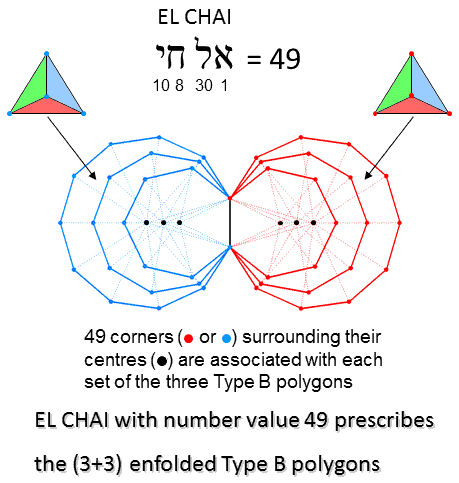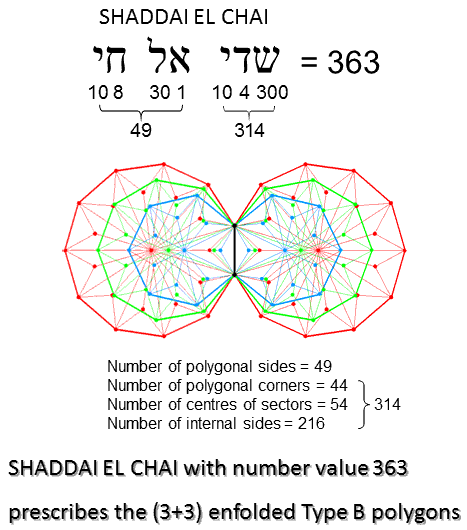<< Previous    1...   9  10    12  13  ...17    Next >>

YesodAccording to Table 2, the 81 triangles in each set of 3 enfolded Type B polygons have 53 corners. 50 corners surround the centres of the 3 polygons. 49 such corners are associated with each set of 3 enfolded polygons. EL CHAI, the Godname of Yesod with number value 49, prescribes the 3 enfolded Type B polygons.The 3 enfolded polygons have 25 sides (24 outside the root edge). The (3+3) enfolded polygons have (1 + 24×2 = 49) sides. 49 is the number value of EL CHAI, the Godname of Yesod. According to Table 2, the 3 enfolded Type B polygons have 186 geometrical elements (183 outside the root edge). The number of geometrical elements in the (3+3) enfolded Type B polygons = 3 + 183×2 = 369. The number of geometrical elements surrounding their centres = 369 − 6 = 363. This is the number value of SHADDAI EL CHAI ("Almighty Living God"), the complete Godname of Yesod. The number 314 of SHADDAI is the number of geometrical elements other than polygonal sides that surround the centres of the 6 polygons. According to Table 2, the 3 enfolded Type A polygons have 157 yods. Outside the root edge, 150 yods surround their centres. Of these, 27 yods are hexagonal yods at the centres of tetractyses. (150−27=123) yods outside the root edge line sides of tetractyses and surround the centres of the 3 polygons. There are (123×2=246) such boundary yods outside the root edge. 246 is the number value of Gabriel, the Archangel of Yesod.

 << Previous    1...   9  10    12  13  ...17    Next >>

Home